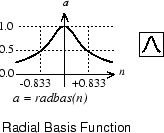## Graph and Symbol## Syntax

```A = radbas(N,FP) ```

## Description

`radbas` is a neural transfer function. Transfer functions calculate a layer’s output from its net input.

`A = radbas(N,FP)` takes one or two inputs,

 `N` `S`-by-`Q` matrix of net input (column) vectors `FP` Struct of function parameters (ignored)

and returns `A`, an `S`-by-`Q` matrix of the radial basis function applied to each element of `N`.

## Examples

Here you create a plot of the `radbas` transfer function.

```n = -5:0.1:5; a = radbas(n); plot(n,a) ```

Assign this transfer function to layer `i` of a network.

```net.layers{i}.transferFcn = 'radbas'; ```

## Algorithms

```a = radbas(n) = exp(-n^2) ```

## Version History

Introduced before R2006a